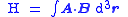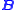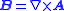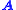xMagnetic helicityEncyclopedia
In plasma physics, magnetic helicity is the extent to which a magnetic field
Magnetic field
A magnetic field is a mathematical description of the magnetic influence of electric currents and magnetic materials. The magnetic field at any given point is specified by both a direction and a magnitude ; as such it is a vector field.Technically, a magnetic field is a pseudo vector;...

"wraps around itself". It is a generalization of the topological concept of linking number
In mathematics, the linking number is a numerical invariant that describes the linking of two closed curves in three-dimensional space. Intuitively, the linking number represents the number of times that each curve winds around the other...

to the differential quantities required to describe the magnetic field. As with many quantities in electromagnetism, magnetic helicity (which describes magnetic field lines) is closely related to fluid mechanical helicity (which describes fluid flow lines).

If magnetic field lines follow the strands of a twisted rope
Rope
A rope is a length of fibres, twisted or braided together to improve strength for pulling and connecting. It has tensile strength but is too flexible to provide compressive strength...

, this configuration would have nonzero magnetic helicity; left-handed ropes would have negative values and right-handed ropes would have positive values.

Formally,whereis the magnetic field strength;is the vector potential
Vector potential
In vector calculus, a vector potential is a vector field whose curl is a given vector field. This is analogous to a scalar potential, which is a scalar field whose negative gradient is a given vector field....

ofMagnetic helicity is a conserved quantity
Conservation law
In physics, a conservation law states that a particular measurable property of an isolated physical system does not change as the system evolves....

. It is conserved in electromagnetic fields, even when magnetic reconnection
Magnetic reconnection
Magnetic reconnection is a physical process in highly conducting plasmas in which the magnetic topology is rearranged and magnetic energy is converted to kinetic energy, thermal energy, and particle acceleration...

dissipates energy
Energy
In physics, energy is an indirectly observed quantity. It is often understood as the ability a physical system has to do work on other physical systems...

. The concept is useful in solar dynamics
Sun
The Sun is the star at the center of the Solar System. It is almost perfectly spherical and consists of hot plasma interwoven with magnetic fields...

and in hydromagnetic dynamo theory. Magnetic helicity is a gauge-dependent quantity, becausecan be redefined by adding a gradient to it (gauge transformation). However, for perfectly conducting boundaries or periodic systems without a net magnetic flux, the magnetic helicity is gauge invariant. A gauge-invariant relative helicity has been defined for volumes with non-zero magnetic flux on their boundary surfaces If the magnetic field is turbulent and weakly inhomogeneous a magnetic helicity density and its associated flux can be defined in terms of the density of field line linkages.